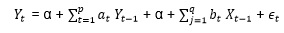# Importance of Granger causality test

By Divya Dhuria,Priya Chetty & Saptarshi Basu Roy Choudhury on September 18, 2018

Granger causality is a method to examine the causality between two variables in a time series. “Causality” is related to cause and effect notion, although it is not exactly the same. It is a statistical concept which is based on the prediction. If X variable’s Granger causes Y, then past values of X should contain information that helps in predicting Y. In more simpler language; if X is influenced by the lagged values of both X and Y, then Y Granger causes X. Similarly, if variation in Y is impacted by its lag and the lagged values of X, then X Granger causes Y.

When Y Granger cause X and X Granger causes Y, this is known as bi-directional Granger causality. If only one Granger causes other, it is uni-directional Granger causality. If both the variables are independent of each other then there is no causality.

## Equations for Granger causality

The equations for Granger causality are as follows:Where ε and n are random disturbances; serially uncorrelated with zero mean and unit variance. α, β a1,a2, .., ap, b1, b2, .., bp are parameters to be estimated.

## The hypothesis for checking causality

The hypothesis for checking the causality using Granger Causality test is as follows:

Null hypothesis: lagged x-values do not explain the variation in y {(x(t) doesn’t Granger-cause y(t)}

Alternative hypothesis: lagged x-values explain the variation in y.

(x(t) Granger-cause y(t))

It helps in investigating the patterns of correlation by using empirical datasets. In FDI study, Granger causality is used to check the robustness of results and to detect the nature of the causal relationship between FDI and GDP.